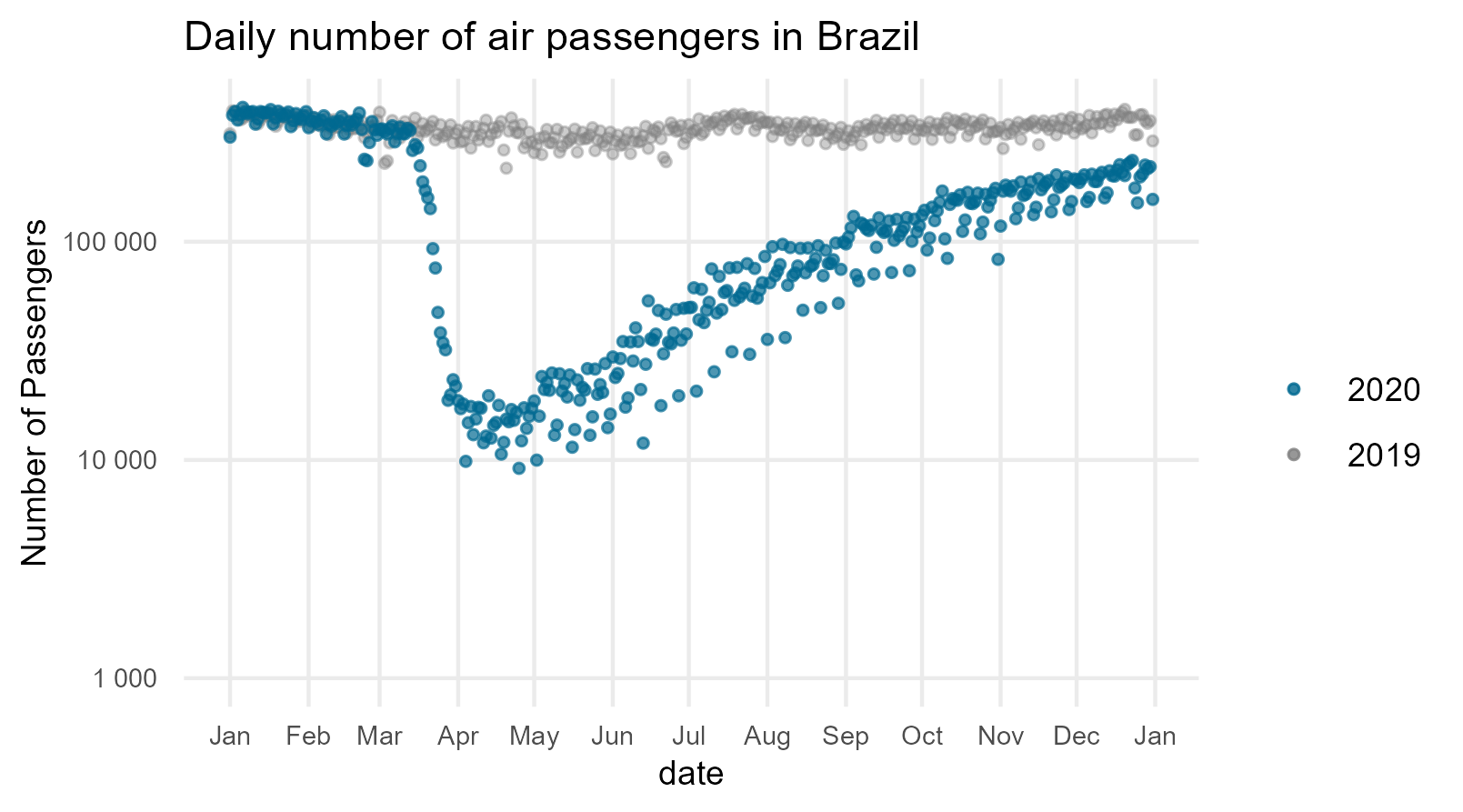# Flights data

The flight data in the flightsbr package is downloaded from Brazil’s Civil Aviation Agency (ANAC). The data includes detailed information on every international flight to and from Brazil, as well as domestic flights within the country. The data include flight-level information of airports of origin and destination, flight duration, aircraft type, payload, and the number of passengers, and several other variables.

Now we can load some libraries we’ll use in this vignette:

library(flightsbr)
library(data.table)
library(ggplot2)

# in a given **month* of a given **year** (yyyymm)
df_201506 <- read_flights(date=201506, showProgress = FALSE)

# in a given year (yyyy)
df_2015 <- read_flights(date=2015, showProgress = FALSE)

If you know already what data columns you need, you can pass a vector with their names to select parameter and read_flights() will only load those columns. This will make the function a bit faster.

df_201506 <- read_flights(date=201506,
showProgress = FALSE,
select = c('id_empresa', 'nr_voo', 'dt_partida_real',
'sg_iata_origem' , 'sg_iata_destino'))

head(df_201506)

The package makes it easy to compare daily number of passengers across different years. In the example below we compare daily number of air passengers in Brazil in 2019 and 2020. This gives us a glimpse in the impact of COVID-19 on Brazilian aviation, similarly to study of Bazzo, Braga and Pereira (2021).

# download flights data
df_2019 <- read_flights(date=2019, showProgress = TRUE)
df_2020 <- read_flights(date=2020, showProgress = TRUE)

# count daily passengers
count_2019 <- df_2019[, .(total_pass = sum(nr_passag_pagos, na.rm=TRUE)) , by = dt_partida_real]
count_2020 <- df_2020[, .(total_pass = sum(nr_passag_pagos, na.rm=TRUE)) , by = dt_partida_real]

# reformat date
count_2019 <- count_2019[ between(dt_partida_real, as.Date('2019-01-01'), as.Date('2019-12-31')) ]
count_2020 <- count_2020[ between(dt_partida_real, as.Date('2020-01-01'), as.Date('2020-12-31')) ]

count_2019[, date := paste0("2030-", format(dt_partida_real, "%m-%d"))]
count_2019[, date := as.IDate(date, format="%Y-%m-%d") ]

count_2020[, date := paste0("2030-", format(dt_partida_real, "%m-%d"))]
count_2020[, date := as.IDate(date, format="%Y-%m-%d") ]

# plot
fig <- ggplot() +
geom_point( data= count_2019, aes(x=date, y=total_pass, color='gray50'), alpha=.4, size=1) +
geom_point( data= count_2020, aes(x=date, y=total_pass, color='#006890') , alpha=.7, size=1) +
scale_y_log10(name="Number of Passengers",
labels = unit_format(unit = ""), limit=c(1000,NA)) +
scale_x_date(date_breaks = "1 months", date_labels = "%b") +
labs(subtitle ='Daily number of air passengers in Brazil', color = "Legend") +
scale_color_identity(labels = c("2020", "2019"), name = "", guide = "legend") +
theme_minimal() +
theme(panel.grid.minor = element_blank(),
axis.text = element_text(size = 7),
axis.title=element_text(size=9),
plot.background = element_rect(fill='white', colour='white'))